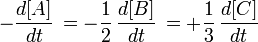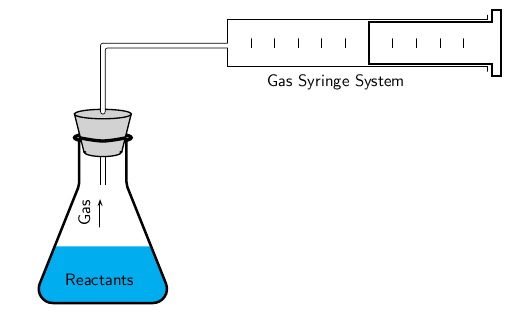## Measuring Reaction Rates

#### Learning Objective

• Produce rate expressions when given chemical reactions and discuss methods for measuring those rates

#### Key Points

• Reaction rate is calculated using the formula rate = Δ[C]/Δt, where Δ[C] is the change in product concentration during time period Δt.
• The rate of reaction can be observed by watching the disappearance of a reactant or the appearance of a product over time.
• If a reaction produces a gas such as oxygen or carbon dioxide, there are two ways to measure the reaction rate: using a gas syringe to measure the gas produced, or calculating the reduction in the mass of the reaction solution.
• If the reaction produces a precipitate, the amount formed can be used to determine reaction rate by measuring how long it takes for the forming precipitate to obscure the visibility of a cross through a conical flask.

#### Terms

• reaction rateHow fast or slowly a reaction takes place.
• productA chemical substance formed as a result of a chemical reaction.
• gas syringeAn item of laboratory equipment used to withdraw a volume of gas from a closed chemical system for measurement and/or analysis.

## Reaction Rate

The rate of a reaction is usually observed by watching the disappearance of a reactant or the appearance of a product within a given time period. Take the chemical reaction:

$A + 2B \rightarrow 3C$

Here, the rate of appearance of product C in time interval Δt is:

$\text{average rate}=\frac{\Delta C}{\Delta t}$

The concentration of C, [C], is usually expressed in moles/liter. This is the average rate of appearance of C during the time interval Δt. The limit of this average rate as the time interval becomes smaller is called the rate of appearance of C at time t, and it is the slope of the curve of [C] versus t at time t. This instantaneous slope, or rate, is written $\frac{d[C]}{dt}$. Since one molecule of A and two molecules of B are consumed for every three molecules of C that are produced, the rates of disappearance and appearance of these chemical species are different, but related.Rates of disappearance and appearance of chemical speciesThis expression relates the rates of disappearance and appearance of chemical species in the reaction A + 2B –> 3C.

## Measuring Reaction Rate

How the rate of a reaction is measured will depend on what the reaction is and what product forms. The following examples describe various ways to measure the rate of a reaction.

## Reactions That Produce Gases Such as Oxygen or Carbon Dioxide

Hydrogen peroxide decomposes to produce oxygen:

$2H_2O_2(aq)\rightarrow 2H_2O(l)+O_2(g)$

The volume of oxygen produced can be measured using the gas syringe method. The gas collects in the syringe, pushing out against the plunger. The volume of gas that has been produced can be read from the markings on the syringe. This change in volume can be converted to a change in concentration ($\Delta [C]$), and dividing this by the time of the reaction ($\Delta t$) will yield an average reaction rate.Gas syringe methodIn a reaction that produces a gas, the volume of the gas produced can be measured using the gas syringe method.

## Changes in Mass

The rate of a reaction that produces a gas can also be measured by calculating the mass loss as the gas forms and escapes from the reaction flask. This method can be used for reactions that produce carbon dioxide or oxygen, but are not very accurate for reactions that give off hydrogen because the mass is too low to be accurately measured. Measuring changes in mass may also be suitable for other types of reactions.

## Precipitation Reactions

In a reaction in which a precipitate is formed, the amount of precipitate formed in a period of time can be used as a measure of the reaction rate. For example, when sodium thiosulphate reacts with an acid, a yellow precipitate of sulfur is formed. This reaction is written as follows:

$Na_2S_2O_3(aq)+2HCl(aq)\rightarrow 2NaCl(aq)+SO_2(aq)+H_2O(l)+S(s)$

One way to estimate the rate of this reaction is to carry out the investigation in a conical flask and place a piece of paper with a black cross underneath the bottom of the flask. At the beginning of the reaction, the cross will be clearly visible when you look into the flask. However, as the reaction progresses and more precipitate is formed, the cross will gradually become less clear and will eventually disappear altogether. By using a stopwatch to time how long it takes for the cross to disappear, and then massing the amount of precipitate formed during this time, an average reaction rate can be calculated. Note that it is not possible to collect the SO2 gas that is produced in the reaction because it is highly soluble in water.